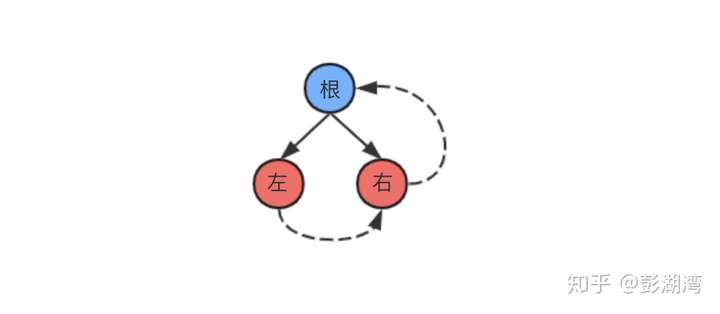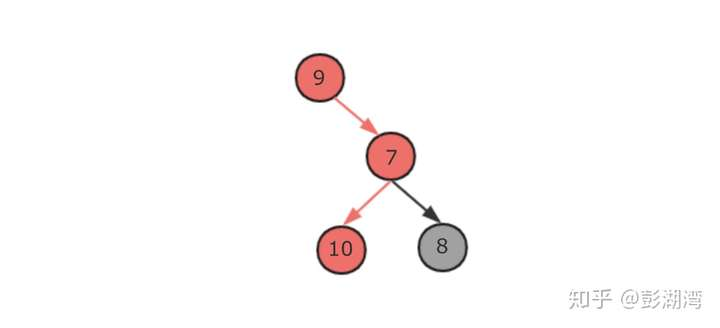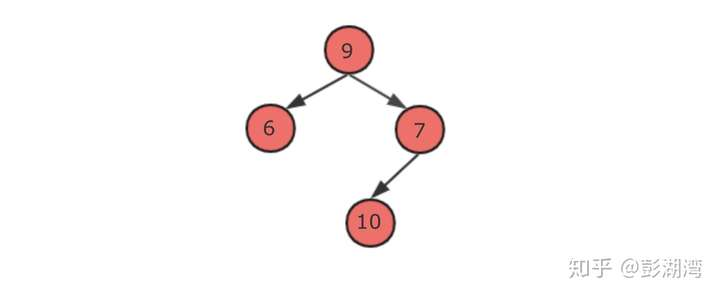# JS刷算法题：二叉树

## Q1.翻转二叉树（easy）

示例：

4
/   \
2     7
/ \   / \
1   3 6   9

4
/   \
7     2
/ \   / \
9   6 3   1

/**
* Definition for a binary tree node.
* function TreeNode(val) {
*     this.val = val;
*     this.left = this.right = null;
* }
*/
/**
* @param {TreeNode} root
* @return {TreeNode}
*/
var invertTree = function(root) {
// 编码
};

var invertTree = function(root) {
traveral(root);
return root;
};

function traveral(node) {
if (node === null) return;
traveral(node.left);
traveral(node.right);
const temp = node.right;
node.right = node.left;
node.left = temp;
}

## Q2.二叉树的右视图(middle)

输入: [1,2,3,null,5,null,4]

1            <---
/   \
2     3         <---
\     \
5     4       <---

1            <---
/   \
2     3         <---
\
5             <---

/**
* Definition for a binary tree node.
* function TreeNode(val) {
*     this.val = val;
*     this.left = this.right = null;
* }
*/

/**
* @param {TreeNode} root
* @return {number[]}
*/
var rightSideView = function(root) {
// 编码
}

1.我们可以设置一个队列存放依次遍历的节点对象。

2.使用两层循环

• 内层循环通过不断出队列的方式遍历当前层的节点，同时通过左右链接收集下一层节点

• 外层循环判断队列长度>0时就继续运行，从而实现逐层迭代

3.在每次内层循环中获取最右端的非空节点

var rightSideView = function(root) {
if (!root) return [];
const queue = [];
const arrRS = [];
// 先保存根结点，也就是第一层二叉树
queue.push(root);
while (queue.length > 0) {
// 将队列长度先保存到一个变量里面
// 表示的是上一层的节点的数量
let length = queue.length;
let temp = null;
// 遍历上一层节点，将它们的子节点加入队列中，收集得到二叉树的下一层
for (let i = 0; i < length; i++) {
// 出队列，并获得返回的父节点
const node = queue.shift();
// 每次都用当前节点的val覆盖temp
// temp最后会等于当前层最右的一个非空节点的val值
if (node.val) temp = node.val;
// 收集当前节点的左节点和右节点，从而得到下一层
if (node.left) queue.push(node.left);
if (node.right) queue.push(node.right);
}
// 收集每一层的最右节点
arrRS.push(temp);
}
return arrRS;
};

## Q3.二叉树中的最大路径和(difficult)

示例1:

1
/ \
2   3

-10
/ \
9  20
/  \
15   7

/**
* Definition for a binary tree node.
* function TreeNode(val) {
*     this.val = val;
*     this.left = this.right = null;
* }
*/
/**
* @param {TreeNode} root
* @return {number}
*/
var maxPathSum = function(root) {
// 编码
};

1.整体思路：通过后序遍历，自底向上计算。2.路径的单一性： 当一个节点是只是作为一个中间节点，而不是一个根节点的时候：左节点和右节点只能选择一个作为经过的路径。 因为路径是“单一”的而不是“分叉”的3.根节点的连接性：当一个节点作为根节点的时候，它可以将两个子树的路径连接起来4. 对于两个子节点的累加值A，B，分3种情况讨论

• A>0,B>0: 选择Math.max(A,B)作为经过路径

• A>0,B<0: 选择A作为经过路径

• A<0,B>0: 选择B作为经过路径

• A<0,B<0: A,B都不选

1. 后序遍历，自底向上计算

2. 对于每个节点，假设它是根结点，计算它联合两个子树路径后的最大值

3. 对于每个节点，假设它是中间节点，选择两条中较大的一条子树作为路径

4. 对于2，3分上面的四种情况进行分别处理

// 1.考虑全为负数的情况
// 2.考虑当前节点为负的情况
let max = Number.MIN_VALUE;
var maxPathSum = function(root) {
max = root.val;
traveral(root);
return max;
};

function traveral(node) {
if (node === null) return 0;
const a = traveral(node.left);
const b = traveral(node.right);
let v = node.val;
if (a >= 0 && b >= 0) {
max = Math.max(max, v + a + b);
v += Math.max(a, b);
} else if (a >= 0) {
max = Math.max(max, v + a);
v += a;
} else if (b >= 0) {
max = Math.max(max, v + b);
v += b;
}
return v;
}

function TreeNode(val) {
this.val = val;
this.left = this.right = null;
}



posted @ 2020-02-11 12:19  彭湖湾  阅读(...)  评论(...编辑  收藏